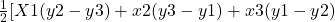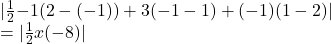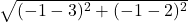## On a coordinate plane, triangle A B C is shown. Point A is at (negative 1, 1), point B is at (3, 2), and points C is at (negative 1, negativ

Question

On a coordinate plane, triangle A B C is shown. Point A is at (negative 1, 1), point B is at (3, 2), and points C is at (negative 1, negative 1)
If line segment BC is considered the base of triangle ABC, what is the corresponding height of the triangle?

0.625 units

0.8 units

1.25 units

1.6 units

in progress 0
6 months 2021-08-24T23:57:45+00:00 2 Answers 0 views 0

D. ( LAST ONE ) 1.6 UNITS

Step-by-step explanation:

IF (x₁,y₁) ,(x₂,y₂) AND (x₃,y₃) ARE THE VERTICES OF THE TRIANGLE.

THEN THE AREA OF THE TRIANGLE ISHERE x₁= -1,y₁=1 ,x₂=3,y₂=2, and x₃= -1,y₃=-1

THEN THE AREA OF THE TRIANGLE IS=4 square units

BC is base of the triangle.

Then the length of BC is == 5 UNITS

Let the height of the triangle be x.

We know that

The area of triangle is

1/2 x base x height

= (1/2x5xX) square units

According to the problem,

1/2 x 5 x X=4

–  X =  4×2/5

X=1.6 UNITS

Therefore the height of the triangle is 1.6 units.